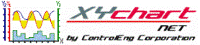XY Chart NET 3 Control Reference
GetYStackIndex Method

Use this method to obtain the corresponding y-stack graph index for the passed in y-scale index.  This method is only applicable if YStacking is enabled.

Syntax
 [Visual Basic] Function GetYStackIndex(YScaleIndex As Integer) As Integer [C#] int XYChartNETCtl.GetYStackIndex(int YScaleIndex) [C++] int GetYStackIndex(int)

Parameters
YScaleIndex
Index of the y-axis scale.
Example
```' XYChartNETCtl1 is the name of the XY Chart NET control instance placed on the form.

Dim YStackIdx As Integer
Dim YScaleIdx As Integer

With XYChartNETCtl1
For YScaleIdx = 0 To .NumYScales - 1
YStackIdx = .GetYStackIndex(YScaleIdx)

Next YScaleIdx
End With
```
```// XYChartNETCtl1 is the name of the XY Chart NET control instance placed on the form.

int YStackIdx;

for (int i = 0; i < XYChartNETCtl1.NumYScales; i++)
{
YStackIdx = XYChartNETCtl1.GetYStackIndex(i);
}
```
```// XYChartNETCtl1 is the name of the XY Chart NET control instance placed on the form.

int YStackIdx;

for (int i = 0; i < XYChartNETCtl1->NumYScales; i++)
{
YStackIdx = XYChartNETCtl1->GetYStackIndex(i);
}
```